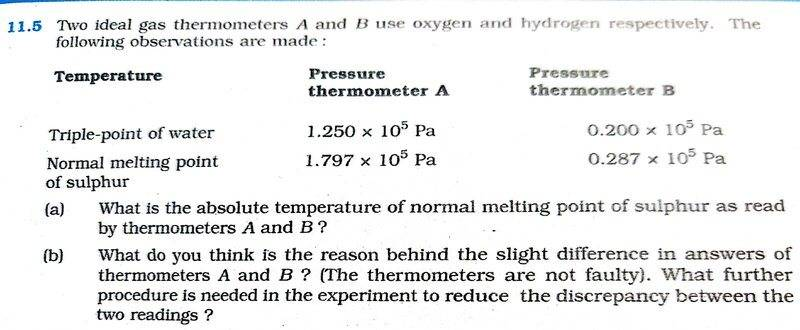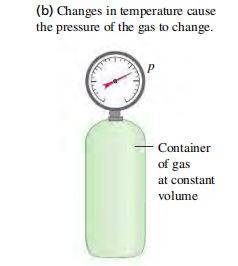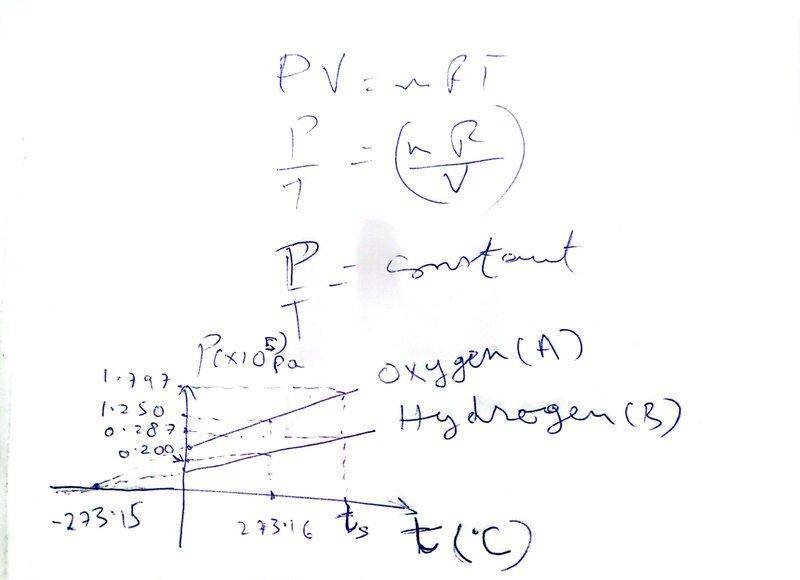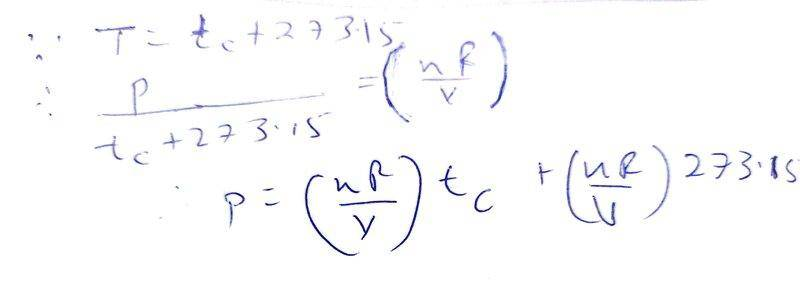# How could the discrepancy be reduced between the two readings?

vcsharp2003
Homework Statement:
The problem is as given in screenshot below. I am unable to correctly answer part (b) of the question.
Relevant Equations:
##\frac {P_1} {T_1} = \frac {P_2} {T_1}## where ##P_1## and ##P_2## denote pressures at temperatures ##T_1## and ##T_2## for a constant volume ideal gasI am able to solve part (a) using the relationship ##\frac {P_1} {T_1} = \frac {P_2} {T_1}##, where ##T_1 = 273.16## since its the triple point of water and ##T_2 =T_s## ##(T_s = ## melting point of sulphur). I use the two readings for thermometer A to get ##P_1## and ##P_2## as mentioned in the first column under Pressure thermometer A.

I use the same approach as above for thermometer B using the readings mentioned in the second column under Pressure thermometer B.

I get correct answers. For thermometer A, ##T_s = 392.69## K and for thermometer B, ##T_s = 391.98## K

For part (b), I am not very clear. The difference in readings could be due to parallax error or observation error by the person using the gas thermometer. But, I'm not sure if there is more to this part (b) answer. Also, I cannot come up with any answer to reduce the difference in ##T_s## determined by thermometers A and B since parallax error or observation error is hard to minimize in an objective manner. In other words, the discrepancy in the two readings in unavoidable and will happen randomly.

Also, my understanding of what a gas thermometer is as shown in the screenshot below.Homework Helper
Gold Member
2022 Award
Non-ideal behaviour of gases?
Expansion of container at the higher pressure?
Expansion of both bottles at the higher temperature?

•vcsharp2003
vcsharp2003
Non-ideal behaviour of gases?
You mean the gases in the two gas thermometers are different and each of them does not exactly behave like an ideal gas, and therefore the difference in their readings. So, we could say that in reality gases approximately act like an ideal gas. Is this explanation correct?

Expansion of container at the higher pressure?
This could happen i. e. volume of gas cylinders would be different for each gas thermometer since pressures are different for these two thermometers and then the volume increase of their containers would also be different. Thus, gases would expand differently in the thermometers making equation P/T=constant an approximation.

Expansion of both bottles at the higher temperature?
Wouldn't this be the same for the two thermometers since they are both subject to the same increase in temperature of ##(T_s - 273.16) ## K? If yes, then this factor shouldn't cause a difference.

Last edited:
vcsharp2003
Non-ideal behaviour of gases?
Also, I have come up with the following analysis for gases in this problem. It seems the slope of the P-tc graph is given by ##\frac {nR} {V}## in this case.

I believe the thermometer B is giving a more accurate reading for ##T_s## since the pressures recorded for thermometer B are very low compared to pressures recorded by thermometer A. Gases behave like ideal gas at low pressures and high temperatures, so applying ##\frac {P} {T}=## constant to thermometer B is more justified.Last edited:
Homework Helper
Gold Member
2022 Award
I believe the thermometer B is giving a more accurate reading for ##T_s## since the pressures recorded for thermometer A are very low compared to pressures recorded by thermometer A.
That sounds reasonable You are dealing with measurement uncertainties of ~0.1% and pressures ~1atm. Deviation from ideal gas behaviour is almost certainly what the question is about.

However, the question specifically asks for some “further procedure”. Is there anything you could do (further measurements) to reduce deviations from ideal gas behaviour?

Also, note the pressure measurements are given to 4 and 3 significant figures. You can’t really justify giving the calculated temperatures to 5 significant figures; I would use 4.

•vcsharp2003
vcsharp2003
However, the question specifically asks for some “further procedure”. Is there anything you could do (further measurements) to reduce deviations from ideal gas behaviour?
I think we need to decrease the number of moles of oxygen in the thermometer A, so it gives lower pressure readings at temperatures of triple point and at melting point of sulphur. That way the oxygen gas will behave more closely to an ideal gas just like thermometer B and we'll get smaller differences in their readings. I am assuming that thermometer B with hydrogen is already at low pressures from the pressure readings mentioned in the original problem.

OR we could decrease the number of moles of oxygen as well as of hydrogen in their thermometers, so they both record low pressures.

Is the above the right approach to decrease the discrepancy between the two thermometers' readings?

Last edited:
vcsharp2003
Also, note the pressure measurements are given to 4 and 3 significant figures. You can’t really justify giving the calculated temperatures to 5 significant figures; I would use 4.
Yes, that's correct. In multiplication and division we take the least number significant digits among the numbers involved. So, for thermometer A calculation we would need to use 4 significant digits and 3 significant digits for thermometer B. Is that right?

Last edited:
Homework Helper
Gold Member
2022 Award
I think we need to decrease the number of moles of oxygen in the thermometer A, so it gives lower pressure readings at temperatures of triple point and at melting point of sulphur. That way the oxygen gas will behave more closely to an ideal gas just like thermometer B and we'll get smaller differences in their readings.
Yes, that is aprobably the "further procedure" they are asking for.

I am assuming that thermometer B with hydrogen is already at low pressures from the pressure readings mentioned in the original problem.

OR we could decrease the number of moles of oxygen as well as hydrogen thermometers, so they both record low pressures.

Is the above the right approach to decrease the discrepancy between the two thermometers' readings?
Yes, reducing both pressure would be even better. Of course, if the pressure is too low, this will increase the fractional uncertainties in the pressure readings. So, best of all, you would use lower pressures for both gases with a higher precision pressure measuring device.

(Another approach would be to use a more accurate equation than the ideal gas equation. Van der Waals equation springs to mind if you have met it. You would need additional information such as the volume.)

•vcsharp2003
Homework Helper
Gold Member
2022 Award
Yes, that's correct. In multiplication and division we take the least number significant digits among the numbers involved. So, for thermometer A calculation we would need to use 4 significant digits and 3 significant digits for thermometer B. Is that right?
Yes. Theat's a sensible use of guidelines. But we are sometimes allowed to 'get away with' an extra significant figure. In this particular problem, I would be tempted to round both temperatures to 4 sig. figs. to make comparison easier.

A better (IMO) approach here might be to assume that pressure readings are ±0.0005x10⁵ Pa, and do a simple error analysis. I got ##T_{S,A}##=(392.7±0.3)K and ##T_{S,B}##=(392.0±1.7)K. The ranges overlap- the "slight disagreement" in temperatures could easily be attributed to random error/limitations of precision. However, it looks like the author of the question didn't consider this and had only deviations from the ideal gas equation in mind.

•vcsharp2003
vcsharp2003
Yes, that is aprobably the "further procedure" they are asking for.

Yes, reducing both pressure would be even better. Of course, if the pressure is too low, this will increase the fractional uncertainties in the pressure readings. So, best of all, you would use lower pressures for both gases with a higher precision pressure measuring device.

(Another approach would be to use a more accurate equation than the ideal gas equation. Van der Waals equation springs to mind if you have met it. You would need additional information such as the volume.)
Would parallax or human observation error also contribute to discrepancy between readings of the two thermometers? I have explained this error in the attempted answer under OP.

Homework Helper
Gold Member
2022 Award
Would parallax or human observation error also contribute to discrepancy between readings of the two thermometers? I have explained this error in the attempted answer under OP.
You can't justify the assumption that parallax error could contribute to the discrepancy.

For example, it's possible that a digital pressure gauge was used, e.g. https://www.instrukart.com/wika-cpg1500-precision-digital-pressure-gauge/

Or, even if an analogue device with a scale w,as used, it might already have included some method to eliminate parallax (e.g. a built-in mirror on the scale behind a pointer).

•vcsharp2003